# Linear Algebra Week 1

Matrices and Vectors

Matrices are 2-dimensional arrays: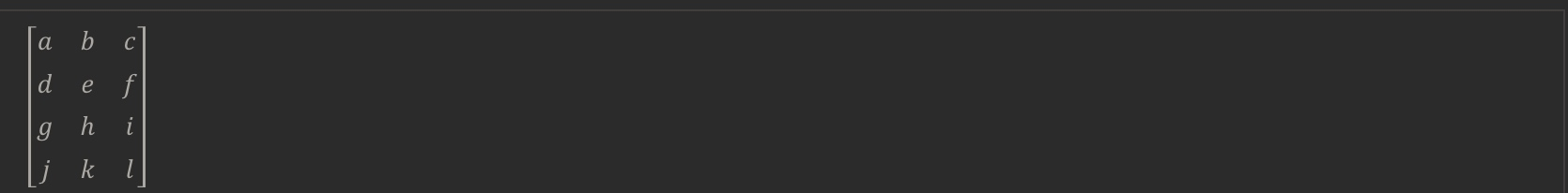The above matrix has four rows and three columns, so it is a 4 x 3 matrix.

A vector is a matrix with one column and many rows:So vectors are a subset of matrices. The above vector is a 4 x 1 matrix.

Notation and terms:
– AijA_{ij}Aij​ refers to the element in the ith row and jth column of matrix A.
– A vector with ‘n’ rows is referred to as an ‘n’-dimensional vector.
– viv_ivi​ refers to the element in the ith row of the vector.
– In general, all our vectors and matrices will be 1-indexed. Note that for some programming languages, the arrays are 0-indexed.
– Matrices are usually denoted by uppercase names while vectors are lowercase.
– "Scalar" means that an object is a single value, not a vector or matrix.
– R refers to the set of scalar real numbers.
– Rn refers to the set of n-dimensional vectors of real numbers.

Run the cell below to get familiar with the commands in Octave/Matlab. Feel free to create matrices and vectors and try out different things.

% The ; denotes we are going back to a new row.
A = [1, 2, 3; 4, 5, 6; 7, 8, 9; 10, 11, 12]

% Initialize a vector
v = [1;2;3]

% Get the dimension of the matrix A where m = rows and n = columns
[m,n] = size(A)

% You could also store it this way
dim_A = size(A)

% Get the dimension of the vector v
dim_v = size(v)

% Now let's index into the 2nd row 3rd column of matrix A
A_23 = A(2,3)

A = “ 1 2 3 4 5 6 7 8 9 10 11 12 “ v = “ 1 2 3 “ m = 4 n = 3 dim_A =

4 3

dim_v =

3 1

A_23 = 6

Addition and subtraction are element-wise, so you simply add or subtract each corresponding element(However, this manipulation must be operate on matrices with same dimension)

In scalar multiplication, we simply multiply every element by the scalar value:

Matrix Vector Multiplication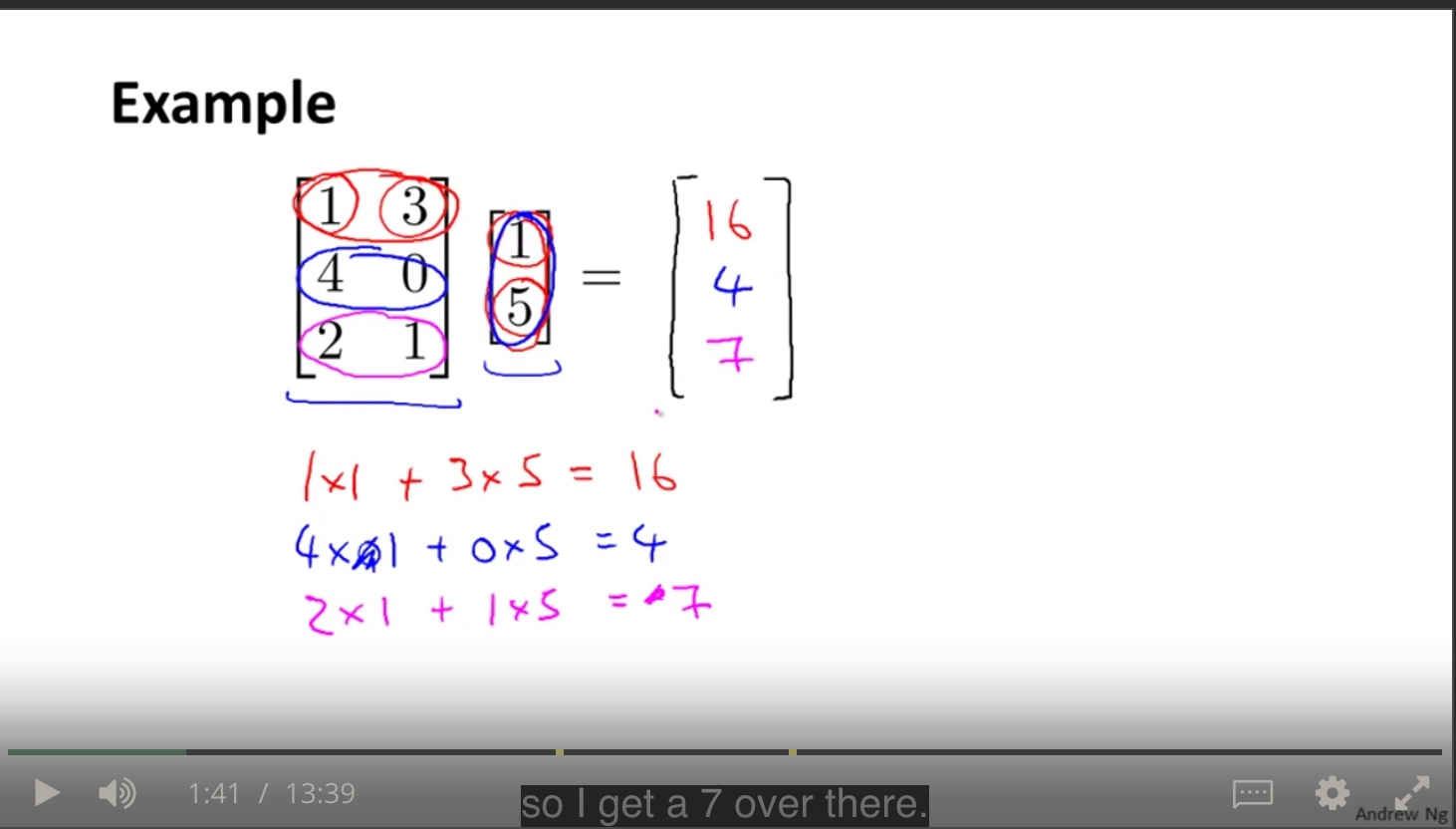trick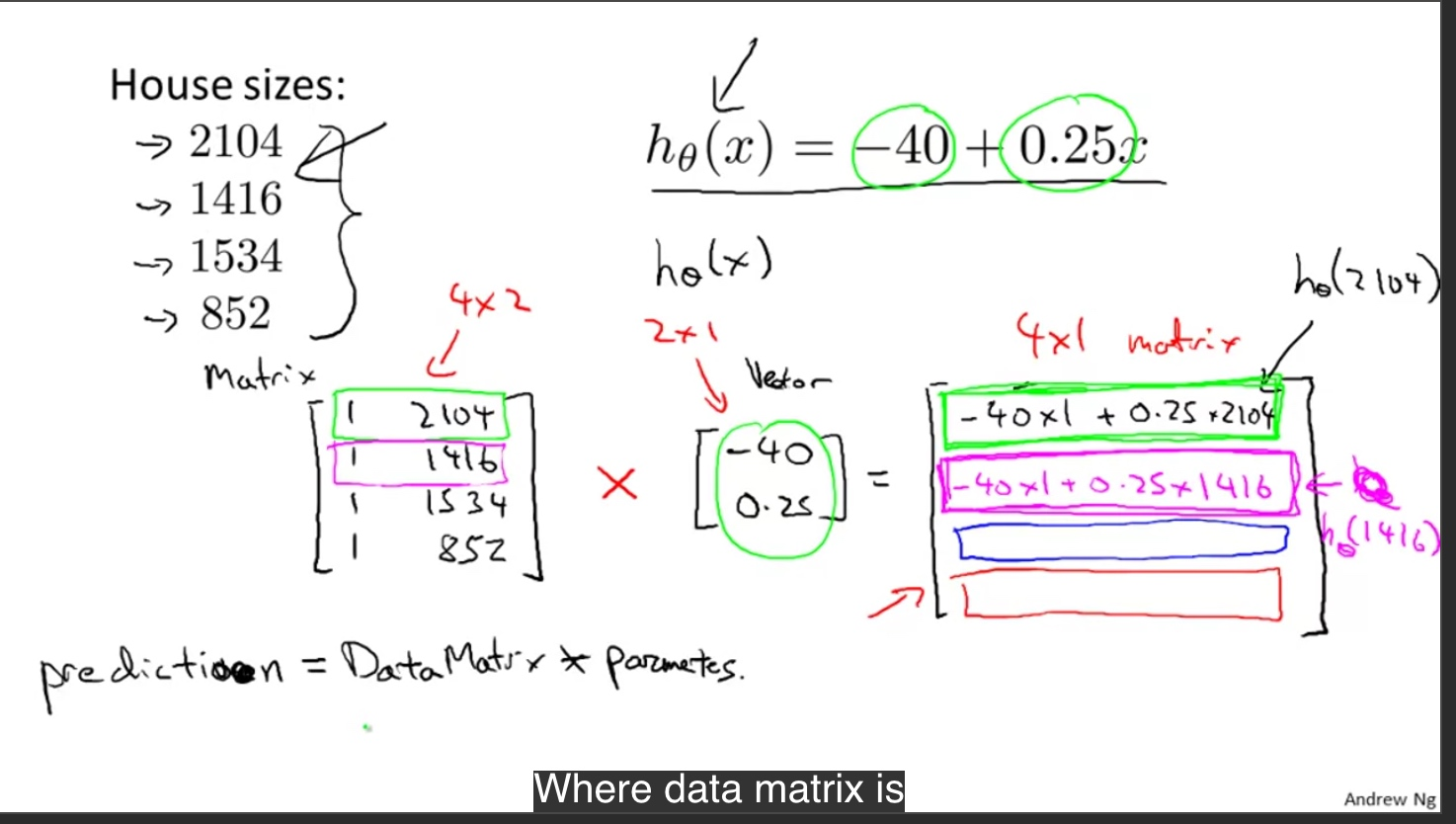Matrix-Vector Multiplication

We map the column of the vector onto each row of the matrix, multiplying each element and summing the result.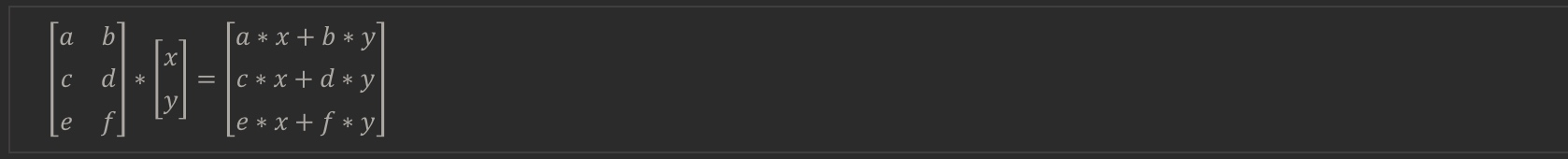The result is a vector. The number of columns of the matrix must equal the number of rows of the vector.

An m x n matrix multiplied by an n x 1 vector results in an m x 1 vector.

Below is an example of a matrix-vector multiplication. Make sure you understand how the multiplication works. Feel free to try different matrix-vector multiplications.

% Initialize matrix A
A = [1, 2, 3; 4, 5, 6;7, 8, 9]

% Initialize vector v
v = [1; 1; 1]

% Multiply A * v
Av = A * v

Matrix Matrix Multiplication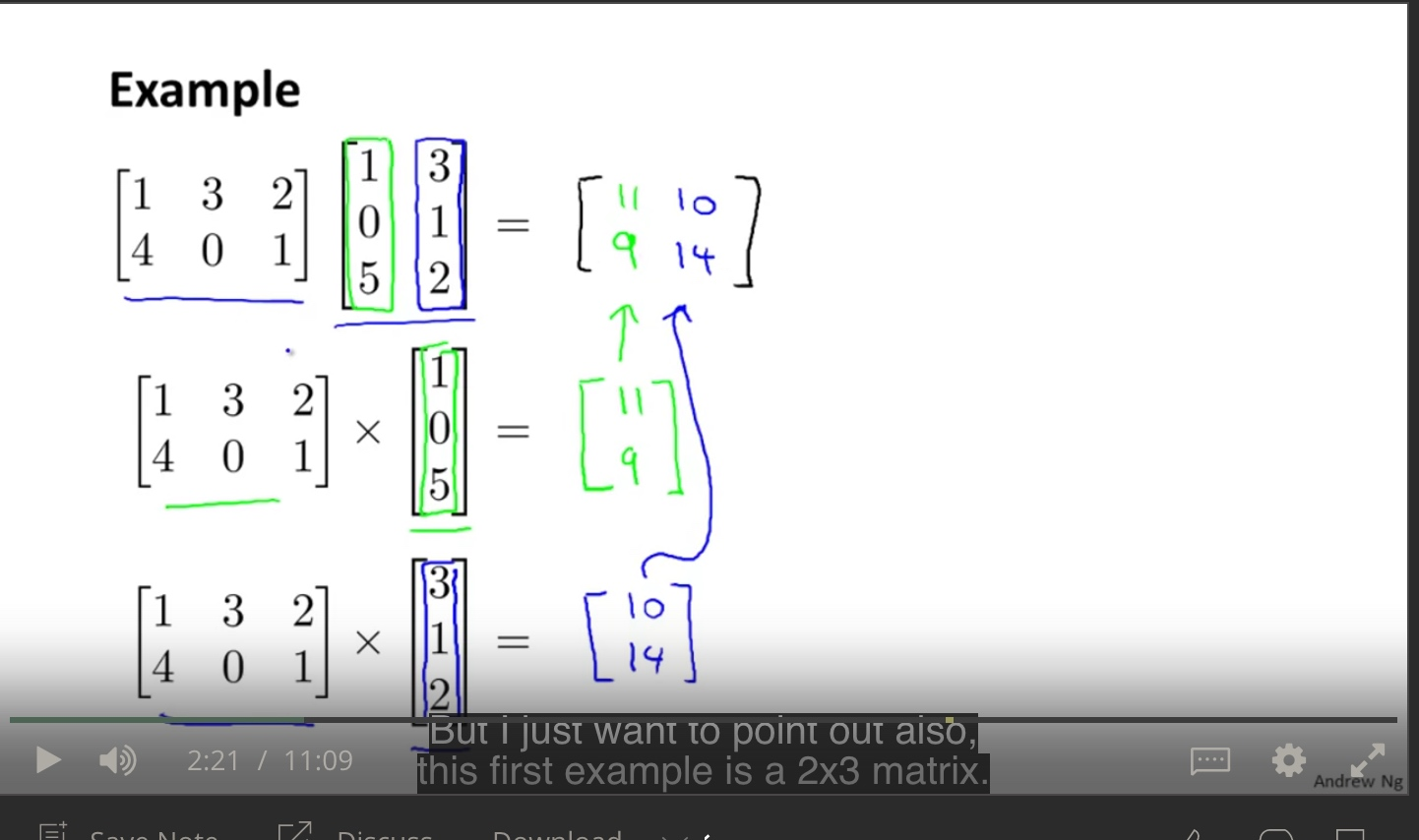mechanism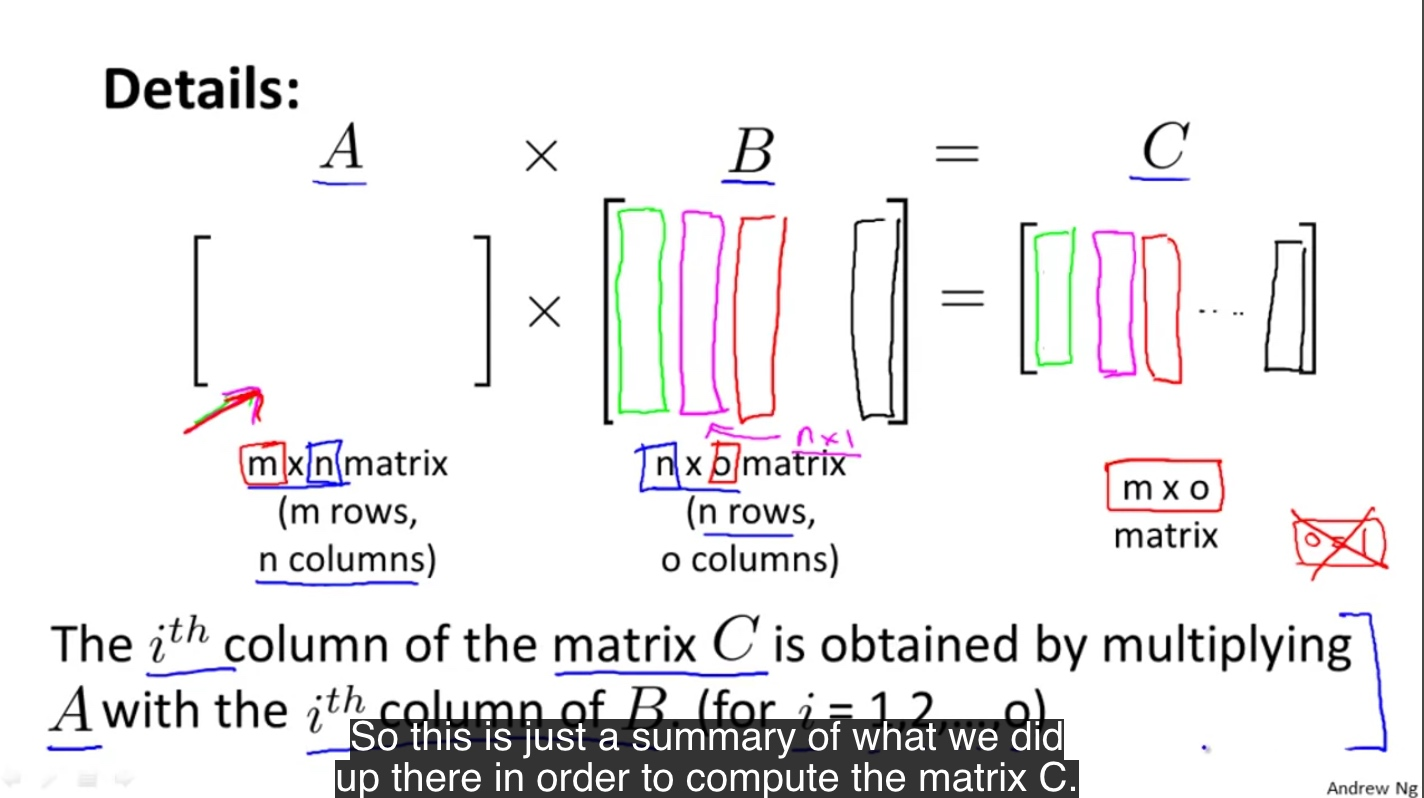trick